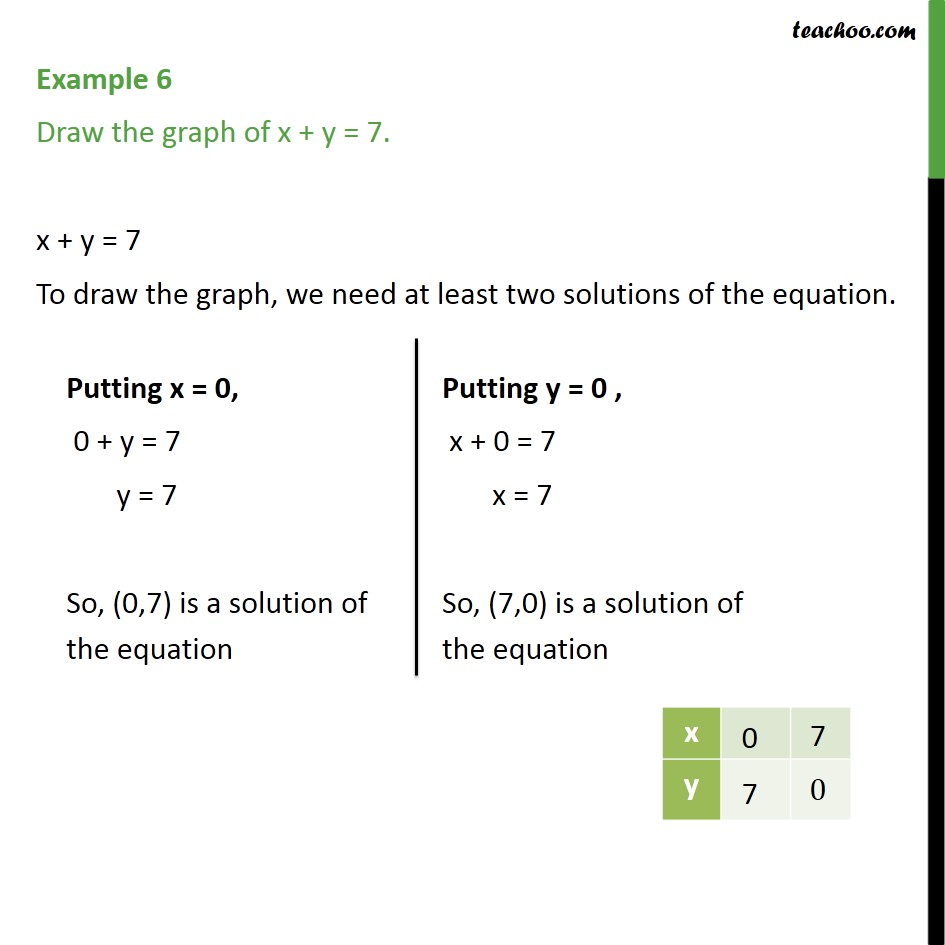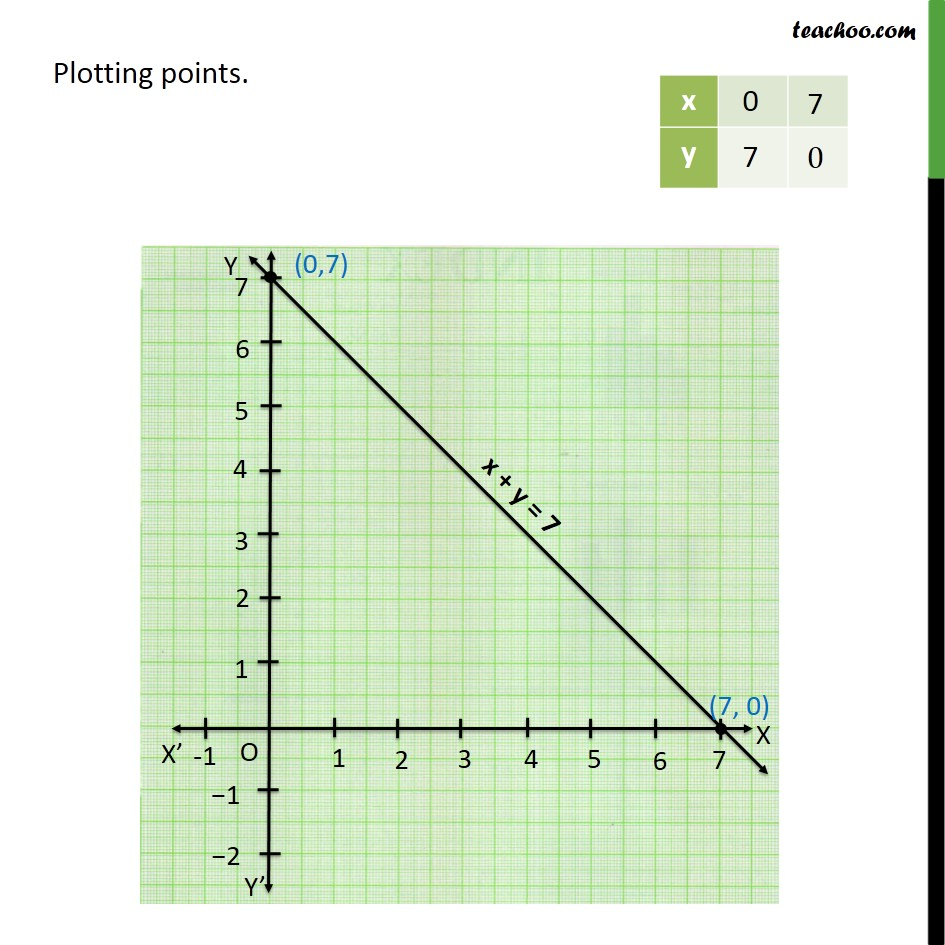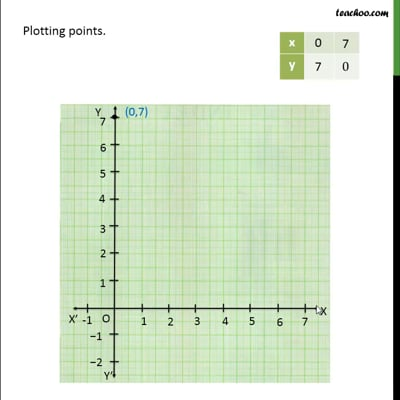Examples

Chapter 4 Class 9 Linear Equations in Two Variables
Serial order wiseThis video is only available for Teachoo black users

Solve all your doubts with Teachoo Black (new monthly pack available now!)

### Transcript

Example 6 Draw the graph of x + y = 7. x + y = 7 To draw the graph, we need at least two solutions of the equation. Putting x = 0, 0 + y = 7 y = 7 So, (0,7) is a solution of the equation Putting y = 0 , x + 0 = 7 x = 7 So, (7,0) is a solution of the equation Plotting points